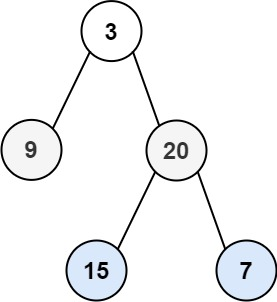0103. 二叉树的锯齿形层序遍历

# 0103. 二叉树的锯齿形层序遍历#

• 标签：树、广度优先搜索、二叉树
• 难度：中等

## 题目大意 #

• 锯齿形层序遍历：从左往右，再从右往左进行下一层遍历，以此类推，层与层之间交替进行。

• 示例 1：1 2  输入：root = [3,9,20,null,null,15,7] 输出：[,[20,9],[15,7]] 
• 示例 2：
 1 2  输入：root =  输出：[] 

## 解题思路 #

### 思路 1：广度优先搜索 #

1. 使用列表 order 存放锯齿形层序遍历结果，使用整数 odd 变量用于判断奇偶层，使用双端队列 level 存放每层元素，使用列表 queue 用于进行广度优先搜索。
2. 将根节点放入入队列中，即 queue = [root]
3. 当队列 queue 不为空时，求出当前队列长度 $s_i$，并判断当前层数的奇偶性。
4. 依次从队列中取出这 $s_i$ 个元素。
1. 如果当前层为奇数层，如果是奇数层，则从 level 末尾添加元素。
2. 如果当前层是偶数层，则从 level 头部添加元素。
3. 然后将当前元素的左右子节点加入队列 queue 中，然后继续迭代。
5. 将存储当前层元素的 level 存入答案列表 order 中。
6. 当队列为空时，结束。返回锯齿形层序遍历结果 order

### 思路 1：代码 #

  1 2 3 4 5 6 7 8 9 10 11 12 13 14 15 16 17 18 19 20 21 22 23 24 25  import collections class Solution: def zigzagLevelOrder(self, root: TreeNode) -> List[List[int]]: if not root: return [] queue = [root] order = [] odd = True while queue: level = collections.deque() size = len(queue) for _ in range(size): curr = queue.pop(0) if odd: level.append(curr.val) else: level.appendleft(curr.val) if curr.left: queue.append(curr.left) if curr.right: queue.append(curr.right) if level: order.append(list(level)) odd = not odd return order 

### 思路 1：复杂度分析 #

• 时间复杂度：$O(n)$。其中 $n$ 是二叉树的节点数目。
• 空间复杂度：$O(n)$。